### Data handling - Solutions

CBSE Class –VI Mathematics

NCERT Solutions
Chaper 9 Data handling (Ex. 9.1)

Question 1. In a mathematics test the following marks were obtained by 40 students. Arrange these marks in a table using tally marks.
8, 1, 3, 7, 6, 5, 4, 4, 2, 4, 9, 5, 3, 7, 1, 6, 5, 2, 7, 7, 3, 8, 4, 2, 8, 9, 5, 8, 6, 7, 4, 5, 6, 9, 6, 4, 4, 6, 6
(a) Find how many students obtained marks equal to or more than 7?
(b) How many students obtained marks below 4?

 Marks Tally Marks No. of students 1.2.3.4.5.6.7.8.9.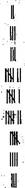233767543

(a) Twelve students
(b) Eight students

Question 2.Following is the choice of sweets of 30 students of Class VI.

(a) Arrange the names of sweets in a table using tally marks.
(b) Which sweet is preferred by most of the students?

 Sweets Tally Marks No. of students ladooBarfiJalebiRasgulla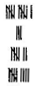11379 30

(b) Ladoo. Because 11 students prefer to eat.

Question 3.Catherine threw a dice 40 times and noted the number appearing each time as shown below:

1, 3, 5, 6, 6, 3, 5, 4, 1, 6, 2, 5, 3, 4, 6, 1, 5, 5, 6, 1, 1, 2, 2, 3, 5, 2, 4, 5, 5, 6, 5, 1, 6, 2, 3, 5, 2, 4, 1, 5

Make a table and enter the data using tally marks. Find the number that appeared.

(a) The minimum number of times.
(b) The maximum number of times.
(c) Find those numbers that appear an equal number of times.

 Numbers Tally Marks How many times? 1.2.3.4.5.6.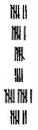7654117

(a) The minimum number of times =4
(b) The maximum number of times = 5
(c) 1 and 6

Question 4. Following pictograph shows the number of tractors in five villages: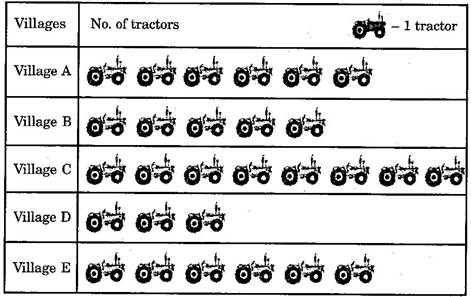Observe the pictograph and answer the following questions:

(i) Which village has the minimum number of tractors?
(ii) Which village has the maximum number of tractors?
(iii) How many more tractors does village C have as compared to village B.
(iv) What is the total number of tractors in all the five villages?

(ii) Village C
(iii) 3
(iv) 28

Question 5.The number of girl students in each class of a co-educational middle school is depicted by the pictograph.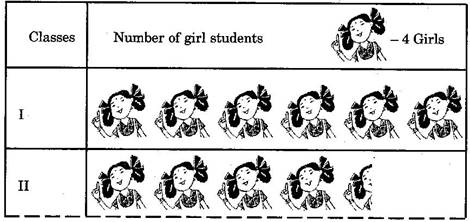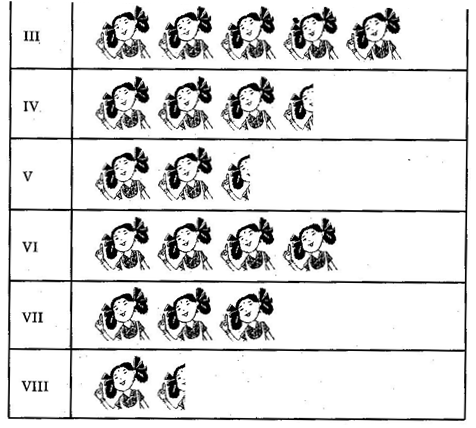Observe this pictograph and answer the following questions:

(a) Which class has the minimum number of girl students?

(b) Is the number of girls in class VI less than the number of girls in class V?

(c) How many girls are there in class VII?

(b) No

(c) 3 x 4 = 12 girls

Question 6.The sale of electric bulbs on different days of a week is shown below: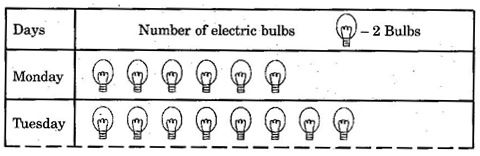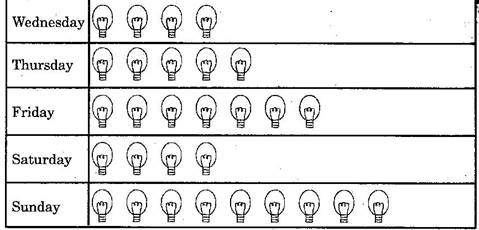What can be concluded from the above pictograph?

Answer: (a) Number of bulbs sold on Monday are 12. Similarly, number of bulbs sold on other days can be found.

(b) Maximum number of bulbs were sold on Sunday.

(c) Same number of bulbs were sold on Wednesday and Saturday.

(d) Then minimum number of bulbs were sold on Wednesday and Saturday.

(e) The total number of bulbs sold in the given week were 86.

Question 7.In a village six fruit merchants sold the following number of fruit baskets in a particular season: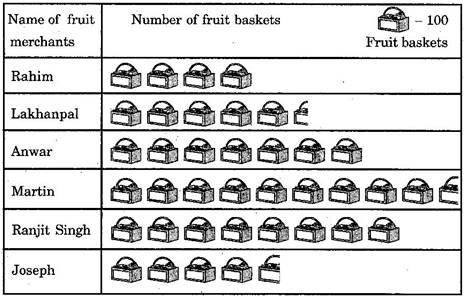Observe this pictograph and answer the following questions:

(a) Which merchant sold the maximum number of baskets?

(b) How many fruit baskets were sold by Anwar?

(c) The merchants who have sold 600 or more number of baskets are planning to buy a godown for the next season. Can you name them?